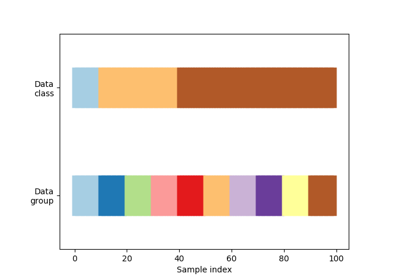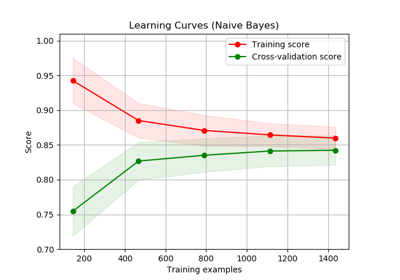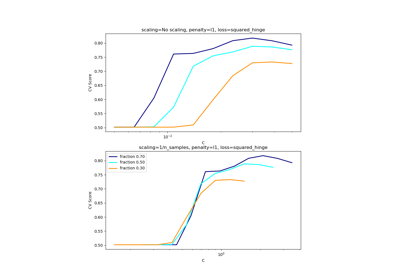sklearn.model_selection.ShuffleSplit¶

class sklearn.model_selection.ShuffleSplit(n_splits=10, test_size=None, train_size=None, random_state=None)[source]

Random permutation cross-validator

Yields indices to split data into training and test sets.

Note: contrary to other cross-validation strategies, random splits do not guarantee that all folds will be different, although this is still very likely for sizeable datasets.

Read more in the User Guide.

Parameters: n_splits : int, default 10 Number of re-shuffling & splitting iterations. test_size : float, int, None, default=None If float, should be between 0.0 and 1.0 and represent the proportion of the dataset to include in the test split. If int, represents the absolute number of test samples. If None, the value is set to the complement of the train size. If train_size is also None, it will be set to 0.1. train_size : float, int, or None, default=None If float, should be between 0.0 and 1.0 and represent the proportion of the dataset to include in the train split. If int, represents the absolute number of train samples. If None, the value is automatically set to the complement of the test size. random_state : int, RandomState instance or None, optional (default=None) If int, random_state is the seed used by the random number generator; If RandomState instance, random_state is the random number generator; If None, the random number generator is the RandomState instance used by np.random.

Examples

>>> import numpy as np
>>> from sklearn.model_selection import ShuffleSplit
>>> X = np.array([[1, 2], [3, 4], [5, 6], [7, 8], [3, 4], [5, 6]])
>>> y = np.array([1, 2, 1, 2, 1, 2])
>>> rs = ShuffleSplit(n_splits=5, test_size=.25, random_state=0)
>>> rs.get_n_splits(X)
5
>>> print(rs)
ShuffleSplit(n_splits=5, random_state=0, test_size=0.25, train_size=None)
>>> for train_index, test_index in rs.split(X):
...    print("TRAIN:", train_index, "TEST:", test_index)
...
TRAIN: [1 3 0 4] TEST: [5 2]
TRAIN: [4 0 2 5] TEST: [1 3]
TRAIN: [1 2 4 0] TEST: [3 5]
TRAIN: [3 4 1 0] TEST: [5 2]
TRAIN: [3 5 1 0] TEST: [2 4]
>>> rs = ShuffleSplit(n_splits=5, train_size=0.5, test_size=.25,
...                   random_state=0)
>>> for train_index, test_index in rs.split(X):
...    print("TRAIN:", train_index, "TEST:", test_index)
...
TRAIN: [1 3 0] TEST: [5 2]
TRAIN: [4 0 2] TEST: [1 3]
TRAIN: [1 2 4] TEST: [3 5]
TRAIN: [3 4 1] TEST: [5 2]
TRAIN: [3 5 1] TEST: [2 4]

Methods

 get_n_splits(self[, X, y, groups]) Returns the number of splitting iterations in the cross-validator split(self, X[, y, groups]) Generate indices to split data into training and test set.
__init__(self, n_splits=10, test_size=None, train_size=None, random_state=None)[source]
get_n_splits(self, X=None, y=None, groups=None)[source]

Returns the number of splitting iterations in the cross-validator

Parameters: X : object Always ignored, exists for compatibility. y : object Always ignored, exists for compatibility. groups : object Always ignored, exists for compatibility. n_splits : int Returns the number of splitting iterations in the cross-validator.
split(self, X, y=None, groups=None)[source]

Generate indices to split data into training and test set.

Parameters: X : array-like, shape (n_samples, n_features) Training data, where n_samples is the number of samples and n_features is the number of features. y : array-like, shape (n_samples,) The target variable for supervised learning problems. groups : array-like, with shape (n_samples,), optional Group labels for the samples used while splitting the dataset into train/test set. train : ndarray The training set indices for that split. test : ndarray The testing set indices for that split.

Notes

Randomized CV splitters may return different results for each call of split. You can make the results identical by setting random_state to an integer.

Examples using sklearn.model_selection.ShuffleSplit¶Visualizing cross-validation behavior in scikit-learnPlotting Learning CurvesScaling the regularization parameter for SVCs